International Journal of Applied Mathematics and Theoretical Physics
Volume 2, Issue 4, October 2016, Pages: 57-63

Functional Basic Units of Physics and Reference Frames That Preserve Maxwell’s Equations

Steven D. Deines

Donatech Corporation, Inc., Fairfield, USA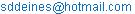Steven D. Deines. Functional Basic Units of Physics and Reference Frames That Preserve Maxwell’s Equations. International Journal of Applied Mathematics and Theoretical Physics. Vol. 2, No. 4, 2016, pp. 57-63. doi: 10.11648/j.ijamtp.20160204.16

Received: August 12, 2016; Accepted: November 9, 2016; Published: December 12, 2016

Abstract: This paper briefly highlights that the basic time unit as the International System second is shorter than the original Universal Time second, which causes the International Atomic Time to run faster than Universal Time. This paper also discusses that the mole, candela, and ampere are functional definitions due to their dependence on other basic physical quantities in the internationally accepted list of fundamental quantities of physics. Particularly, electrical current in amperes is not fundamental concerning charge of electrons or protons. The ampere combines charge and time units, which makes it a functional quantity—not fundamental. Also, the definition of the ampere underscores a paradox with inertial frames. The expected forces between current-carrying wires that are moving can be explained only by an absolutely stationary frame. Maxwell’s electromagnetic equations are based on empirical results over the past two centuries. The Lorentz force, which is velocity dependent, violates Newton’s second law and the Equivalence Principle concerning inertial frames. If a Newtonian force, such as gravity, accelerates all points parallel and equally at each instant of time within the domain of a reference frame, then that frame is mathematically equivalent to an absolutely stationary frame. The speed of light is guaranteed to be a universal constant as well as all other electromagnetic constants within an absolutely stationary frame, which is mathematically equivalent for laboratories. Any slight variation of Newtonian forces within a laboratory is virtually undetectable with electromagnetic phenomena. Thus, Maxwell’s equations are valid only within an absolutely stationary reference frame.

Keywords: Second, Time, Candela, Ampere, Kelvin Degree, Charge, Mole, Maxwell’s Equations, Lorentz Force

Contents

1. Introduction

Physics is one of many quantitative sciences that involve measurements, experiments and a system of theoretical principles that attempt to explain the results of experiments in the simplest model. It uses the scientific method to correlate many observed facts gained from the experimental results against various theoretical models so that predictions can be made and tested with more precise experiments. By collecting facts through careful observation, a discovery of relationships is made so generalizations can be made into fundamental principles called physical laws.

As physics is quantitative, measurements of physical quantities consist of a number and units of various phenomena. Any physical quantity used in the formulation of laws and principles must be operationally defined by specifying the procedures used to measure the quantity. For convenience, certain physical quantities have fundamental units and are measured in arbitrary, but internationally accepted methods through examples or basic procedures. All other physical quantities are defined in terms of those fundamental quantities and are derived quantities using these basic units.

The General Conference for Weights and Measures (CGPM in French) has accepted seven terms as fundamental basic units of science: second, meter, kilogram, Kelvin, candela, mole and ampere. The Système Internationale (SI) second is defined as the duration of 9192631770(20) periods of the radiation corresponding to the transition between the two hyperfine levels of the ground state of the cesium 133 atom, where the digits in parentheses designate the 1suncertainty in cycles from Ephemeris Time (ET) at 1957.0 from the original 3-year calibration . The lunar ET second was accepted by the International Committee of Weights and Measures in 1956 as the standard time unit . The precision reached 9 significant digits in the definition of the ET second, which is identical for all practical purposes with the present SI second . Deines and Williams identified the main factor of divergence between Universal Time (UT)1 and International Atomic Time (TAI) timescales is the mean UT second (based originally on Earth’s rotation) is minutely longer than the SI second that is the basic time unit in TAI . This was due to the omission of time dilation required by relativity in the Improved Lunar Ephemeris (ILE) theory  that defined time intervals between observed lunar positions. The ILE accurately accounted for Earth’s rotational deceleration as documented by Deines and Williams . The time spans were used to calibrate the oscillations emitted from excited cesium atoms as the predicted time transpired between lunar observations. That atomic calibration was conducted by US Naval Observatory (USNO) and the National Physics Laboratory (NPL) between 1955 and 1958. The scientific community later accepted that result  as the definition for the SI second for the unit in TAI. However, this same team that meticulously calibrated TAI using the ILE model reported that the mean UT second was longer than the ET (equivalently SI) second in the observed 1955-1958 data [6, 7]. No relativity effects were included in the ILE theory , which causes the SI second (i.e. identical to the undilated ET second) to be shorter than the mean UT second of 1958 by 2.468E-8 s/s . The virtually linear divergence between UT and TAI as maintained by leap second insertions between 1958 and 1998 is within 0.2% of this size difference [2, 5]. There are at least 8 other timing discrepancies that are explained by this size difference between the original UT second and the SI second . This issue may seem trite, but astronomers rely on the original UT timescale to replicate ancient astronomical observations or make future long-term calculations of heavenly bodies for dynamical astronomy, such as eclipses, conjunctions, spacecraft landings, etc. Thus, the current time definition needs to be reviewed for consistency to compensate this difference in these basic time units, but that debate is outside the scope of this paper.

The physical meter stored by the Bureau International des Poids et Mesures (BIPM) in Paris has been replaced by the definition that the meter length is the path travelled by light in a vacuum during the time interval of 1/299792458 of the SI second. If one uses the original UT second instead of the SI second for the definition of the meter, the size difference between different seconds will affect the meter at the eighth significant digit, instead of the ninth significant digit from the definition of the speed of light in a vacuum. This means the definition of the meter can be off by approximately 24.68 nm, depending upon which second is the actual time unit used.

The kilogram is the unit of mass, which is the international prototype of the kilogram maintained by BIPM. Even then, this mass is subject to reversible surface contamination with the air at the rate of roughly 1mg per year, which requires a specific cleaning process prior to using this standard . The utilization of the kilogram is limited to 9 significant figures after a year of its previous cleaning. These three fundamental quantities of time, length, and mass in terms of the international standards of the respective second, meter, and kilogram with Newton’s three classical laws define the MKS system of mechanics.

In thermodynamics, the measurement of thermal energy due to atomic motion is defined by the Kelvin temperature difference with its unit being the Kelvin (or Celsius) degree. The mole is the chemical unit or the amount of substance that contains as many elementary entities as there are atoms in 12 grams of carbon 12 as specified in the periodic chart . Another designated base unit is the candela, which is the luminous power (total light as radiant energy per second weighted by the human eye’s sensitivity to various wavelengths [photopic]) emitted per solid angle (steradian). In electromagnetism, the fundamental unit for electric current is chosen to be the ampere, which is defined in coulombs per second. Technically, all other quantities used in physics can be derived from these designated fundamental units. One can algebraically convert between two physical quantities provided the quantities have identical units before addition or subtraction or can be rescaled by multiplication or division to the same unit before combining the quantities.

The Kelvin degree qualifies as a basic unit of physics for temperature, because it is a physical quantity different from the quantity of heat. The individual movement of atoms, ions and molecules conveys the classical interpretation of kinetic energy, and statistical mechanics interprets the mean thermal energy of all those particles in equilibrium as heat of the object. The principle of thermal equilibrium allows the thermometer’s reading to achieve the same temperature as that of the object. To calibrate a thermometer, two convenient reproducible temperatures were used: the lower fixed point (the ice point by mixing pure ice and water at standard atmospheric pressure) and the upper fixed point (the steam point of pure boiling water at standard atmospheric pressure). Liquid mercury thermometers were used to measure the distance of mercury’s expansion, so that 100 equal spaces designated the Celsius scale (0°C to 100°C) or 180 spaces (32° F to 212° F) for the Fahrenheit scale. Although no practical upper temperature limit exists, the lowest limit of absolute zero is the state that a body of atoms is incapable of giving up any thermal energy . The Kelvin scale has a lowest temperature of absolute zero of 0° K in Celsius-sized degrees based on an ideal gas model with constant pressure , and the Rankine scale begins at 0° R (i.e. ~459.67° F) in Fahrenheit-sized degrees.-This absolute temperature scale only requires one fixed point to determine the size of the degree, and the better fixed point is the triple point of water that all three states coexist at one temperature and pressure, which is assigned the temperature 273.16°K exactly , giving the experimental ice point value of 273.1500° K . The kilocalorie, which is the energy to raise the temperature of one kilogram of water from 14.5°C to 15.5°C , can measure the quantity of heat. Heat and thermal energy have the same relationship as work and mechanical energy. Conservation of thermodynamic energy can be expressed as heat added plus work done equals the increase in thermal energy plus increased mechanical kinetic energy plus the increase in mechanical potential energy of the system. Joule demonstrated that mechanical work done on water raised the temperature of the water by a degree by approximately 4184 J = 1 kcal . Temperature is not a measure of thermal energy, but is a measure of the ability of a hotter body to transfer thermal energy to another cooler body . In summary, the Kelvin degree (or an equivalent type of measure) is a basic unit of physics that is not defined from the other fundamental units of physics.

However, upon closer examination, some of the other accepted basic units of science do not qualify as independent standards of physics. Avogadro proposed the hypothesis that equal volumes of any two gases under the same conditions of temperature and pressure contain equal numbers of molecules , which later became known as Avogadro’s law. When equating an element in grams to its relative atomic mass in the periodic chart, the model is equivalent to Avogadro’s number of atoms that constitute the naturally occurring mix of isotopes for that element. Avogadro’s constant is 6.02214199(47) x 10-23 atoms per mole , where the digits in parentheses represent the 1s uncertainty in the last digits of the given number. So, one mole of the pure isotope of C12 would represent 12.000000 grams of mass, which is roughly equivalent in uncertainty to the international standard of the kilogram that gains mass from surface contamination over time. Thus, Avogadro’s constant is dependent on the international kilogram standard, which makes the mole a derived functional constant.

Photometric equipment is calibrated to the candela to measure the human eye’s sensitivity to light emitted in a particular direction. The accepted model for the human eye is based on an average from various human test subjects that revealed a changing visual sensitivity over the visual spectrum. The 16th General Conference of Weights and Measures (CGPM) in 1979 made the definition of the candela in terms of lumens (total light emitted by a light source in terms of radiant power in units of watts as weighted by the photopic function) per solid angle, but candela is not an independent unit as its definition depends on the watt with the photopic function. Illumination engineers provide recommended lighting to illuminate offices, hotel lobbies, libraries, sidewalks, etc. Photometric measurements are scaled to the selective sensitivity of the human eye, while radiometric measurements use nonselective detectors that are equally responsive to radiant energy of all wavelengths. Although the candela is the illumination unit for people, it is a functionally defined term that is obtained from energy units per time in basic units of time, mass, and length and does not qualify as a basic fundamental physics unit.

In the field of quantum physics, the basic unit of electrical charge of a proton or electron is ±1 charge, which is ±1.602176462(63)E-19 coulomb . An electrical current of one ampere represents a flow of charge at the rate of 1 C/s past any point. To obtain this coulomb unit for electrostatics in the MKS system, one multiplies time with ampere to get an ampere-second. One is struck with the internationally recognized concept that an ampere is a fundamental unit by mixing charge and time units. An ampere has no sign to distinguish charge, because all currents are assumed to be electron flows. By convention, the positive current is always the opposite direction that electrons flow. Coulomb himself measured that the force between two static charges is proportional to the magnitude of the point charges and inversely to the square of the distance between the charges. With force F expressed in newtons (or units of kg m/s2) ,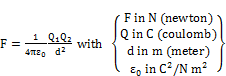(1)

It is considered that e0 is a dimensional constant called the permittivity of free space. To distinguish charges, one arbitrarily assigns the proton’s charge as +1 and the electron’s charge to -1. This immediately proves that an ampere is never a fundamental unit, because the ampere cannot distinguish between a current of positive versus negative charges.

2. Problems with the Ampere Unit

The definition of an ampere  is given as follows:

"The ampere is that constant current which, if maintained in two straight parallel conductors of infinite length, of negligible circular cross section, and placed 1 meter apart in vacuum, would produce between these conductors a force equal to 2 x 10E-7 newtons per meter of length."

The time unit is mixed in the definition of the ampere, which is a functional method to calibrate current. Within today’s technology, one ampere should have far higher precision. To illustrate, atomic clocks have a typical precision of 1E-15 s/s, interferometers with screw length adjustments can easily measure nanometers, but the actual current depends on the measured force between wires. Note the ampere is limited to one significant figure for the force per unit length, and the word "exactly" is missing in the definition. The defined procedure for an ampere relies upon the meter to separate the wires, time unit for accumulated charge, and the force/length in N/m (or in kg/s2). This demonstrates again that an ampere is not an independent unit, but a functional electrical unit that is derived. A fundamental unit for electromagnetism should be the proton or electron charge, which is ±1.602176462(63)E-19 C, respectively. Regardless if the proton or electron is moving or stationary, it still retains the same unit charge in electromagnetism, quantum mechanics, atomic physics, or high energy physics.

Biot and Savart experimentally found that magnetic induction, B, at distance r from a long straight wire carrying a current, I, is proportional to the current and inversely proportional to the distance. The Biot-Savart law [18, 19] in the MKS system for a closed circuit with the proportional constant permeability of free space, μ0, is: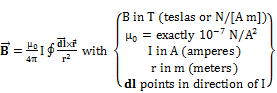(2)

For two straight parallel conductive wires separated by distance d, the Biot-Savart law reduces [12, 18] to: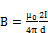(3)

The magnetic force law expresses the force exerted by a closed circuit A with a current IA on a closed circuit B with its current IB. This magnetic force law  is: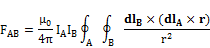By the Biot-Savart law (2), the magnetic force  becomes:(4)

The force per unit length between two infinitely long wires that carry equal current I [12, p. 787], [18, p. 297], [19, p. 173] is: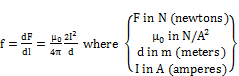(5)

If both wires conduct 1 A of current in the same direction, the force per unit length of wire is attractive by (4); if the wires conduct current in opposite directions, the force per unit length of wire is repulsive by (4). The functional definition for 1 ampere of current moving in each wire is given by (5). Current is not fundamental as listed in the international standard definitions, because current in each wire depends on the fundamental quantities of number of moving charges (nq), time and length for the mean velocity, and mass per unit length of wire to measure the Lorentz force (given in Equation (6) below), which is created by the induced magnetic field from the other wire.

3. Paradox Between Inertial Frames and Electrical Currents

Time plays an essential part of the functional definition of current. Currents are conveyed by electrons moving between atoms on the surface of the conductors, while the interior atoms are electrically shielded from the exterior electromagnetic fields. Consider four separate cases of two thin parallel wires of nearly infinite length and separated by one meter: {1} absolutely stationary Wires 1 and 2 have 1 A currents in opposite directions, {2} absolutely fixed Wires 1 and 2 have 1 A currents moving in the same direction, {3} stationary Wire 1 has a current of 1 A, Wire 2 has an electrostatic surface charge, but Wire 2 is moving collinearly parallel with the same velocity of the current flowing in Wire 1, and {4} stationary Wire 1 has 1 A current, and Wire 2 has its surface electrostatically charged, but no current flows as Wire 2 moves collinearly with the opposite velocity of the current in Wire 1. Using Equations (3) and (4), the infinite wires will generate opposite repulsive forces of 2E-7 N per unit length of wire for case {1}. In case {2}, the same equations predict the infinite wires generate attractive forces of 2E-7 N per unit length of wire.

Consider case {3}. A point charge has an electric field intensity E and, when moving, creates a magnetic inductance B that exerts a force on currents or other moving charged particles. The moving Wire 2 causes the Lorentz force , which is: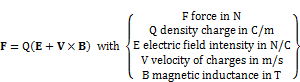(6)

Let Wire 2 be electrostatically charged with a uniform surface density of Q electrons per meter. For simplicity in case {3}, let Wire 2 move collinearly with a velocity V identical to the current velocity in Wire 1, so that QV = 1 ampere past a point in Wire 1’s frame. For Wire 1 in case {3}, the electric field E is contained between each electron and proton pair. No effective E field is conveyed from Wire 1. Although Wire 2 is electrostatic with Q, QE from Wire 2 does not exert a force on the neutral Wire 1. Only the Q(V x B) term contributes in the Lorentz force. Wire 1 has a constant current and generates a stationary magnetic field B1 with equipotentials in cylindrical shapes that are parallel and symmetric along the wire. Wire 2 has no current relative to itself, but the macroscopic motion of the charged Wire 2 makes QV equivalent to a current of 1 ampere in the inertial frame of Wire 1. A magnetic field B2 is generated in the stationary Wire 1 frame by the moving current in Wire 2 passing by in the same direction of Wire 1’s current. By equation (4), Wire 1 experiences an attractive force of 2E-7 N per unit length.

Relative to Wire 2’s inertial frame, the electrostatic charges are stationary with no induced magnet field. Then, IB = 0 in equation (4) relative to Wire 2’s inertial frame. Also, the current in Wire 1 does not exist in Wire 2’s frame and magnetic induction B1 = 0, since Wire 2 is moving externally with the same velocity as the current in Wire 1. So, the Lorentz force on Wire 2 in its inertial frame is F2 = Q(V2 × B1) = Q(0 × 0) = 0. Likewise, the Lorentz force exerted on Wire 1 in the frame of Wire 2 is F1 = Q(V1 × B2) = Q(0 × 0) = 0. However, Newton’s third law requires that Wire 2 must have an attractive force of 2E-7 N per unit length pulling Wire 2 toward Wire 1. Equation (4) predicts zero force in Wire 2’s inertial frame. An observer moving with Wire 2 must sense the force and surmises that equation (4) must be true in a stationary (i.e. laboratory) frame. The observer concludes the surface electrons on Wire 2 are creating an effective current in the laboratory frame (i.e. Wire 1’s frame), and, according to (4), the attractive force indicates that Wire 2 is moving parallel with the current in Wire 1. With the initial conditions (1 A current in Wire 1, the distance between the wires, and the measured attractive force on Wire 2), the observer calculates the magnitude of the velocity of Wire 2 from equation (4). The observer moving with Wire 2 can determine the velocity V relative to the stationary laboratory frame external of Wire 2’s inertial frame by internal measurements. It has always been postulated by the Equivalence Principle that an inertial observer can never determine the external velocity of the observer’s inertial frame relative to an absolutely stationary frame, but this example theoretically contradicts that postulate.

Case {4} also has a similar paradox with its given conditions. Wire 2 moves in the opposite direction of the current electrons on Wire 1 with a velocity V so that QV = 1 ampere past a point in Wire 1’s frame. Equation (4) predicts Wire 1 experiences a repulsive force of 2E-7 N per unit length away from Wire 2. The surface current I1 is moving at twice the speed in Wire 2’s inertial frame than in Wire 1’s frame. The induced magnetic field B1 in Wire 2’s inertial frame is twice the magnitude of the induced B2 of Case 1. However, Wire 2 has no I2 current (i. e. V2 = 0), so the Lorentz force on Wire 2 in its inertial frame is F2 = Q(0× B1) = 0. In the inertial Wire 2 frame, no magnetic field B2 is induced since no current flows on Wire 2, making the predicted Lorentz force on Wire 1 as F1 = Q(V1 × 0) = 0. In the stationary laboratory frame (i.e. Wire 1’s frame), Wires 1 and 2 experience repulsive forces of 2E-7 N/m in Wire 1’s stationary frame, but no force is expected between the wires in Wire 2’s inertial frame, because no movement of charges occurs on Wire 2. This violates the Equivalence Principle that no experiment can detect any intrinsic difference between reference frames in uniform motion with different velocities. The way to avoid this paradox is assume that the force observed in the absolutely stationary frame is also observed in the moving inertial frame.

This second paradox can be resolved if Newton’s third law applies to Wire 1’s result of a Lorentz force from Wire 2, so that Wire 2 has a repulsive force of 2E-7 N/m from Wire 1. The solution of V in F2 = Q(E + V2 × B1) is Wire 2 has the required velocity V in the opposite direction of the current in Wire 1 in the absolutely stationary frame. The solution of B in F1 = Q(E + V × B2) will require that Wire 2 has an B2) will require that Wire 2 has an equivalent current I2 relative to the absolutely stationary frame, which indicates Wire 2 is moving at a speed V, but moving collinearly in the opposite direction of the current in Wire 1. It is also noteworthy that if Q electrons/length were stripped from Wire 2, the test results of Case {3} and {4} would be interchanged as Wire 2 would move positive current. This demonstrates again that the ampere definition fails to distinguish between positive or negative current flows if the ampere was a true fundamental unit of electromagnetism.

The electromagnetic equations must apply to an absolutely stationary frame only. If one assumes Wire 2 is absolutely stationary in Cases {3} and {4} and Wire 1 is moving, there is no net Lorentz force between the wires as there is no effective current I2 in the stationary frame of Wire 2 as the charges are electrostatic. In Case {3}, let Wire 1 move collinearly with a constant velocity V carrying its own current I1 moving at the same velocity V relative to Wire 1. Then, the electrostatic charges on Wire 2 appear to be moving in the opposite direction in Wire 1’s inertial frame of the I1 current, which would imply there should be a Lorentz force of 2E-7 N/m repelling the two wires by Equation (5). If the current I1 was moving in the opposite direction relative to Wire 1 for Case {4}, Equation (5) predicts that there would be a Lorentz force of 2E-7 N/m attracting the two wires in the inertial Wire 1 frame. This is a real paradox. The resolution is obtained only if the electromagnetic equations apply to the absolutely stationary frame of reference and if Newton’s third law applies for either stationary or moving inertial frames.

Of the four known natural forces, only gravity is external to the atomic structure, which contains the strong, weak and electromagnetic forces. Newton himself proved that any Newtonian force, such as gravity, that is applied parallel and equal to all points within a domain of a reference frame will be equivalent to an absolutely stationary frame. After postulating his three laws of mechanical physics, Newton stated in Corollary VI, "If bodies, moved in any manner among themselves, are urged in the direction of parallel lines by equal accelerative forces, they will all continue to move among themselves, after the same manner as if they had not been urged by those forces" . Newton summarized his proof, "For these forces acting equally (with respect to the quantities of the bodies to be moved), and in the direction of parallel lines, will (by Law 2) move all the bodies equally (as to velocity), and therefore will never produce any change in the positions or motions of the bodies among themselves" . By these limitations, Maxwell’s electromagnetic equations are preserved only in absolutely stationary frames. With the precision used in most electrical circuitry, the tiny variation of gravity within the reference frame’s domain (i.e. laboratory) is not a detectable acceleration difference with electromagnetic phenomena to cause any deviation within Maxwell’s equations. Thus, the validity of Maxwell’s equations is only assured within an absolutely stationary reference frame.

A reference frame is inertial if Newton’s laws are valid in that frame (i.e. the same force equation applies in different inertial [nonaccelerated] frames). All inertial frames can have some constant velocity relative to other inertial frames, because the derivative of a constant velocity is zero, which produces no added Newtonian force in the second law of mechanics to change the same Newtonian force equations in another inertial frame. But, electrodynamics has forces that are velocity dependent, such as the Lorentz force. Thus, one must be careful to use absolutely stationary reference frames with Maxwell’s equations. Moving inertial frames do not preserve velocity-dependent forces due to the different velocities between pairs of moving inertial frames. Because the electrical reference frame such as a laboratory in Earth’s gravity is mathematically equivalent for all practical purposes to an absolutely stationary frame, the speed of light is guaranteed to be a universal constant by Maxwell’s equations in a stationary frame, because there is no velocity vector of that frame to add to light’s speed.

4. Conclusion

Of the seven basic physical units (kilogram, meter, second, Kelvin, candela, mole and ampere) accepted by the CGPM, the mole, candela and ampere are not true fundamental quantities because they depend on other physical units. The mole is simply the count of atoms that make up an atomic mass of a specific element in grams relative to the international standard kilogram. The mole is technically equivalent to the international standard kilogram, but it is not independent of the kilogram. The candela has a functional definition that depends on the illumination units of power in watts per solid angle that is scaled to the sensitivity of the human eye. The candela is a practical unit, but it can be derived from other units, similar as the watt is derived from the meter, kilogram and second units. The ampere is defined by a test scenario that relies on other basic units, which demonstrates it is a functional parameter, not a fundamental unit. The unit charge for a proton or electron is a tiny fraction of an ampere-second, but one has to arbitrarily assign the correct unit charge for a proton or electron. This demonstrates the ampere is functional, because the ampere definition makes no allowance for the difference between current flows of protons versus electrons. Also, the atomic or SI second is shorter than the original UT second by 2.468E-8 s/s , which is observable [6,7,9] as that difference is over 10 times larger than the uncertainty of the SI second definition. For example, the divergence between UT and TAI based upon the leap second insertions between January 1958 and December 1998 is within 0.2% of this size difference between the SI and UT seconds [3,5]. The scientific community needs to resolve the time unit issue of the different sizes between the original UT and ET (alias SI) seconds, which cause many timing anomalies .

The ampere scenario is more problematic. The formal definition of an ampere is based on the force per unit length (i.e. 2E-7 N/m) between two thin parallel wires separated by a meter, each carrying an electrical current of 1 ampere. Parallel currents in the wires will have an attractive force; antiparallel currents cause a repulsive force. If the second wire has a uniform electrostatic charge per unit length, but that wire moves collinearly with the same velocity or opposite velocity to the current velocity of charges moving in Wire 1, then Wire 1 experiences the Lorentz forces that created the same forces from the effective current in Wire 2 as before. However, Wire 2 has no effective velocity of charges in the Wire 2 frame, so any observer fixed in the Wire 2 frame should not detect any Lorentz forces interacting between Wire 2 and Wire 1. This paradox is resolved if Newton’s third law applies. If the observer fixed to Wire 2 senses the required opposite force from interacting with absolutely stationary Wire 1, then the solution of the Lorentz force equation with the conditions of 1 ampere of current in Wire 1 with an attractive (or repulsive) force/length and one meter separation between the parallel wires predicts the observer fixed to Wire 2 has an effective velocity parallel (or antiparallel) to the current in Wire 1 in the stationary laboratory (Wire 1’s frame). The Lorentz force and the related Maxwell equations apply mathematically for only absolutely stationary frames of reference. The moving charged wire scenarios demonstrate that an observer fixed with the moving charged wire can determine the external velocity of the moving wire from internal measurements due to interaction of the Lorentz force. So, the postulate that all inertial frames preserve the same force equation is not universal with electromagnetism. The force equations in electromagnetism are preserved only in absolutely stationary frames. Inertial frames have the same force equations when dealing with Newtonian forces, which obey Newton’s second law.

Newton  proved in his Corollary VI that all bodies that are equally accelerated by Newtonian forces (i.e. forces obeying his second law) in parallel lines within a reference frame behave as if no acceleration was applied to the bodies of that reference frame. Essentially, Newton proved mathematically that all Newtonian forces that can accelerate all points universally parallel at each instant within any frame is equivalent physically to absolutely stationary reference frames. All inertial frames preserve the same Newtonian force equation, because the derivative of a constant velocity between inertial frames is zero, which produces no added Newtonian force. However, electrodynamics has forces that are velocity dependent, such as the Lorentz force, which those force equations are not the same between inertial frames. Thus, Maxwell’s equations are valid within absolutely stationary frames. This will guarantee that the speed of light is universal in a stationary frame where Maxwell’s equations remain valid for all practical purposes until experimental precision is increased to detect the subtle deviations from Maxwell’s equations from tiny variations of external Newtonian forces (such as gravity) within the laboratory.

References

1. Markowitz, W., Hall, R. G., Essen, L., and Parry, J. V. L., "Frequency of Cesium in Terms of Ephemeris Time", Physical Review Letters, Aug. 1958, 1, No. 3, p. 105-107.
2. Markowitz, W., "Comparisons of ET(soloar), ET(lunar), UT, and TDT" in The Earth’s Rotation and Reference Frames for Geodesy and Geodynamics, IAU Symposia #128, Babcock, A. K. and Wilkins, G. A. (eds.), (1988) p. 413-418.
3. Deines, S. D. and Williams, C. A., "Time Dilation and the Length of the Second", Astron. J., (2007) 134, 64-70.
4. Improved Lunar Ephemeris 1952-1959, U.S. Government Printing Office, Washington, D. C., (1954), prepared jointly by Nautical Almanac Offices of the United States of America and the United Kingdom.
5. Deines, S. D. and Williams, C. A., "Earth’s Rotational Deceleration: Determination of Tidal Friction Independent of Timescales", Astron. J. (2016) 151, p. 103-115.
6. Essen, L., Parry, J. V. L., Markowitz, W., and Hall, R. (1958) "Variation in the Speed of Rotation of the Earth since June 1955", Nature, 181, 1054.
7. Markowitz, W., "Variations in the Rotation of the Earth: Results Obtained with the Dual-Rate Moon Camera and Photographic Zenith Tubes", Astron. J., (1959) 64, 106-113.
8. McCarthy, D. D. and Seidelmann, P. K., Time—From Earth Rotation to Atomic Physics, Wiley-VCH Verlag GmBH & Co. Weinheim (2009).
9. Deines, S. D. and Williams, C. A., "Two Differently Sized Seconds Explains Many Time Anomalies", submitted to Astron. J., (2016).
10. BIPM website: www.bipm.org/en/measurement-units/
11. Woan, G., The Cambridge Handbook of Physics Formulas, Cambridge University Press, 2003.
12. Shortley, G. and Williams, D., Elements of Physics, 4th ed., Prentice-Hall, Inc., 1965.
13. Kelvin, W., "On an Absolute Thermometeric Scale", Philosophical Magazine, October 1848.
14. "Resolution 3: Definition of the Thermodynamic Temperature Scale", Resolutions of the 10th CGPM, Bureau International des Poids et Measures, 1954.
15. Joule, J. P., "On the Mechanical Equivalent of Heat", Phil. Trans. of the R. Soc. of London, (1850) 140, p. 61-82.
16. Avogrado, L. R. A. C., "Mémoire sur les masses relatives des molécules des corps simples, ou densités présumées de leur gaz, et sur la constitution de quelques-uns de leur composés, pour servir de suite à l'Essai sur le même sujet" (trans. "Note on the Relative Masses of Elementary Molecules, or Suggested Densities of Their Gases, and on the Constituents of Some of Their Compounds"), le Journal de Physique, July 1811.
17. CGPM, The 9th General Conference of Weights and Measures, Resolutions 2 and 7, (1948).
18. Lorrain, P. and Corson, D. R., Electromagnetic Fields and Waves, 2nd ed. (1970) W. H. Freeman and Co., San Francisco.
19. Jackson, J. D., Classical Electrodynamics, 2nd ed., John Wiley and Sons, 1975.
20. Newton, I., Mathematical Principles of Natural Philosophy, 3rd ed., (1725) translated from Latin by Andrew Motte, revised by Florian Cajori, Encyclopedia Britannica, Inc., 21st printing, (1977) LOC 55-10341.

Footnotes

 UT0 is the timescale based on mean solar time from Earth’s rotation as determined by observed meridian transits. Polar wobble affects UT0 at the observer’s latitude. The UT1 timescale is adjusted for polar wobble and synonymous to UT in this paper. The former solar timescale Greenwich Mean Time (GMT) is now called UT.

 Contents 1. 2. 3. 4.
Article ToolsAbstractPDF(217K)